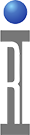When making intermodulation measurements on devices, establishing the appropriate spurious free dynamic range is the primary challenge to a valid measurement. This is done by striking a balance between intermodulation created in the measurement system and the noise floor of the measurement system. When these two are equal the maximum S.F.D.R. is achieved.

Inter-modulation in the measurement system is reduced by attenuation, This fundamental technique will not be discussed in this note as it is widely understood, and common to all measurement systems.

This note improves upon the other side of the equation, that of the measurement system noise floor. Since IPx measurements are generally done with significant attenuation to avoid system created IM products, the desired IM signal is generally close at a very low amplitude.

The receiver in the Ri7100 and Cassini systems, is a two stage down-conversion system. The first stage down-converts to a 21.4 MHz IF, the signal is then filtered to a 7kHz bandwidth, then amplified by an IF amplifier, and the second stage down converts to DC for detection.

There are two mechanisms that will affect the floor of this measurement. First noise, but more importantly in this case, leakages, spurs and offsets. The DC level at the A/Ds in the absence of a signal under test, will be a function of any 21.4 MHz spur on the RX LO, any leakage of 21.4 MHz of the detector board LO into the system and the residual DC offsets of the RX IQ demodulator. This will in effect set a floor for any signal measurement at the exact tuned receiver frequency.

In the diagram below, A simple representation of a measurement of a 1000 MHz signal is shown. That frequency establishes an RX LO frequency of 1021.4 MHz.

the three limiting signals are represented in red. Any signal on the RX LO 21.4 MHz away for the frequency of 1021.4 will show up on top of the desired dut signal. Any leakage for the 21.4 MHz LO will be there as well. After they are all down-coverted to DC they will be adding to the DC offsets of the demodulator. In effect this sets a floor to the measurement but it isn't a "noise" floor.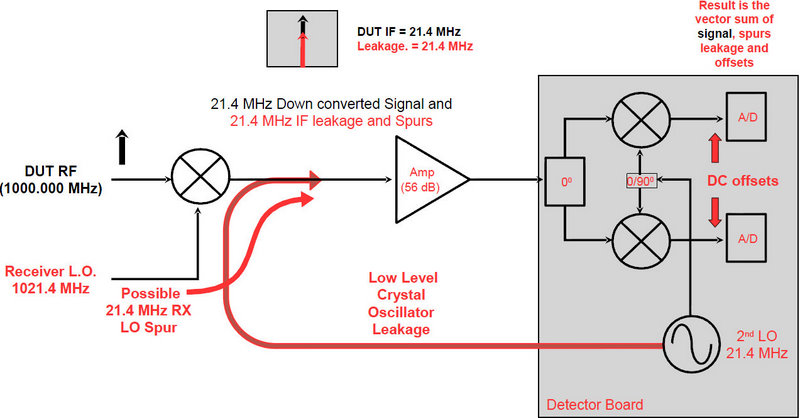We have a way around this spurious measurement floor. The receiver's detector has bandwidth greater than DC and the IF filter's 7kHz Bandwidth can be utilized.

The technique is to offset the set measurement receiver's frequency enough to move away from the DC term and yet keep the desired tone inside the 7kHz IF Bandwidth. A 2 kHz offset is sufficient.
In the example below, the desired is still at 1000 MHz, but in this case the RX is tuned to 1000.002 MHz. This creates two signals in the IF, both the leakages that occur exactly at 21.4 MHz and the desired tone at 21.402 MHz. When they are both mised with the final LO, the 21.4 tones end up at DC and the 21.402 tones show up as a 2 kHz sinusoid.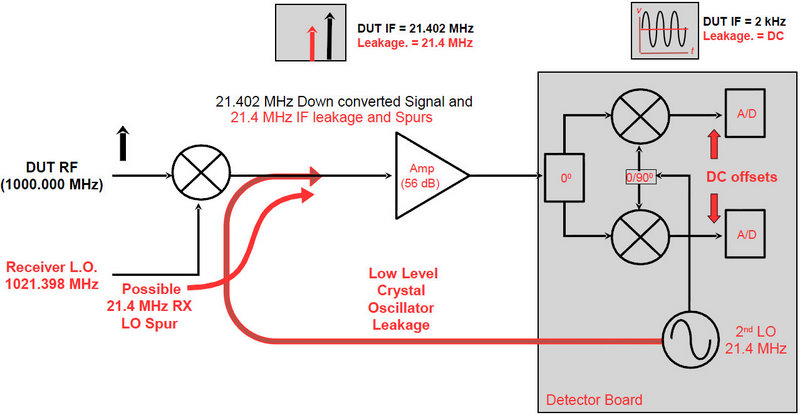Here is a test plan example on the Cassini system the implements the measurement. The Value of A at Freq B calcualtion does a DFT calc on the wave form and extracts the voltage at 2 kHz. When using the value in additional calculations be sure to convert to the appropriate units (power dBm etc)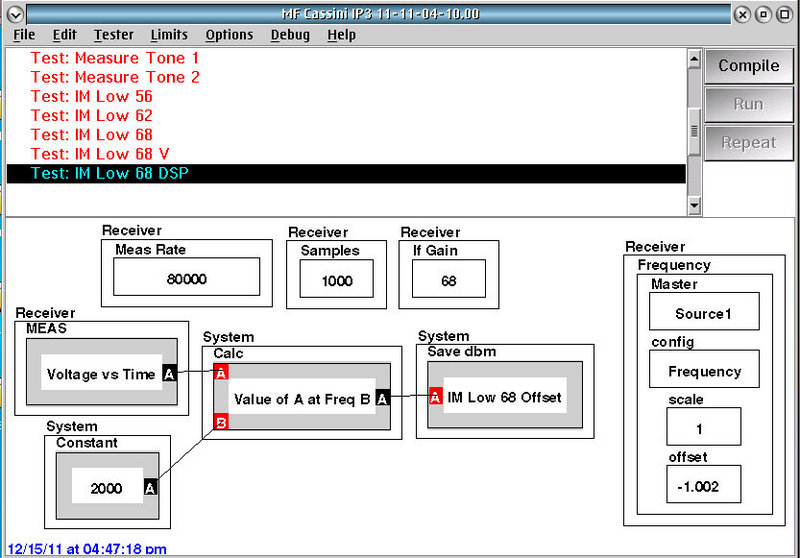Here is an example from the Ri7100. It is worth noting that the in both Cassini and the 7100 the frequency offset function can be used.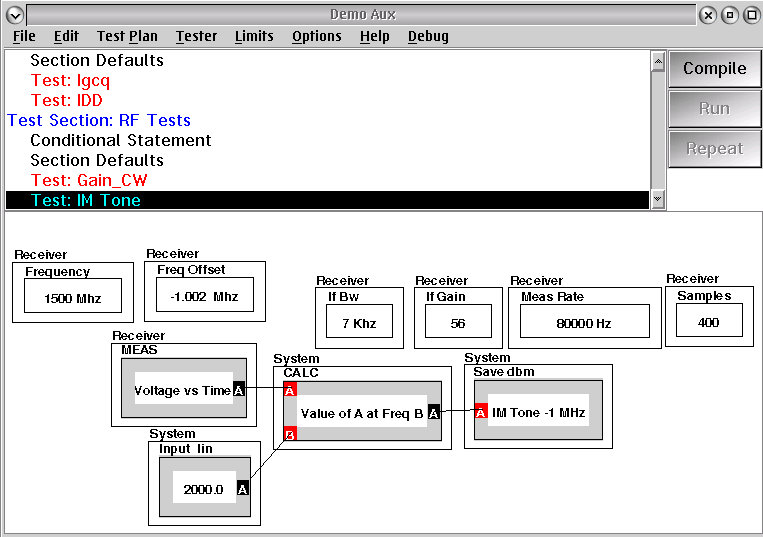Here is a spectral representation from the tester showing both the DC residuals and the desired signal for a reasonably measurable signal (20 dB above the DC spur).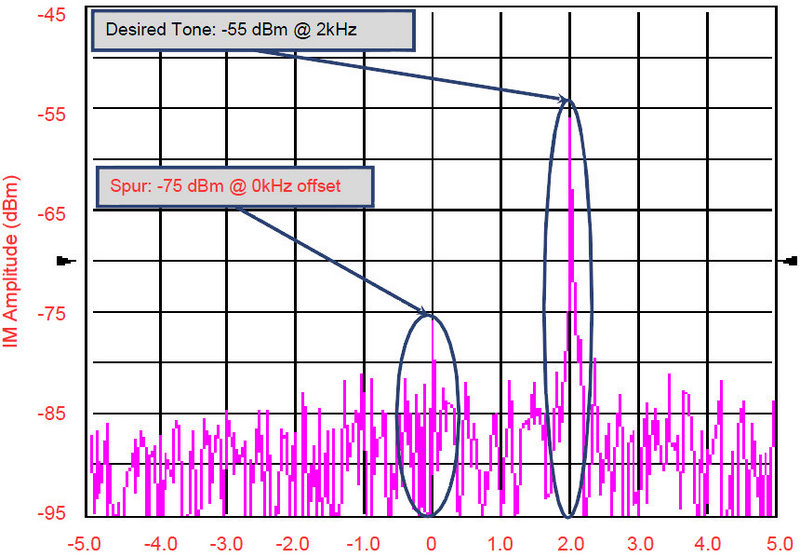In this case the 2 kHz signal is approximately equal to the residual spur. If the tone was at the DC, the vector sum, measurement to measurement would give a rather poor standard deviation (+6, - infinity, log scale)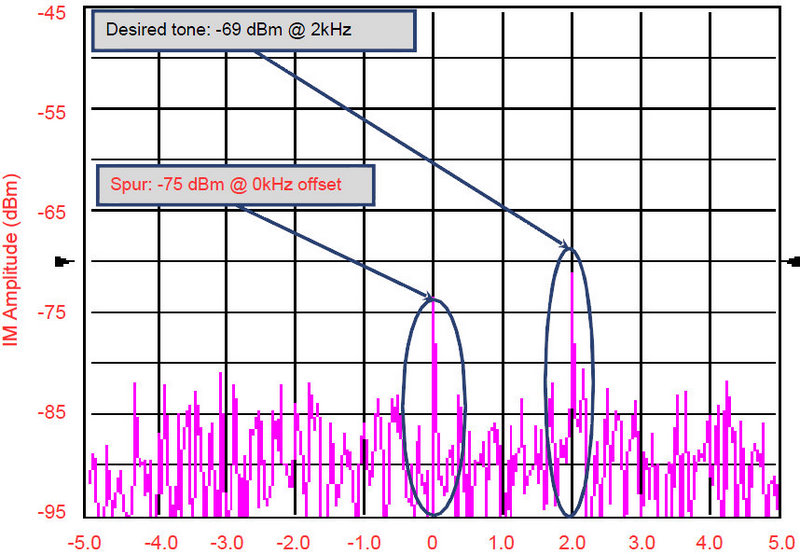In this case the desired tone is actually below the input referenced signal level of the spur. This would not have been mensurable at all in the conventional case.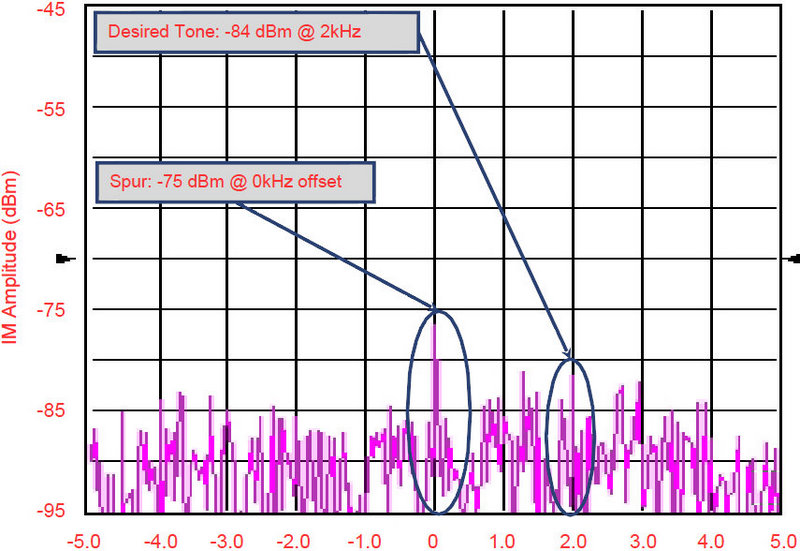Here is a graphical representation of measuring an IM tone, both in the offset mode and directly tuned to the signal.
When directly tuned to the signal the apparent level of the desired tone hits a floor, that in effect is the DC residual at the detectors.. When measuring with the offset approach, the signal can be measured significantly lower in power, until it reaches the real noise floor of the system.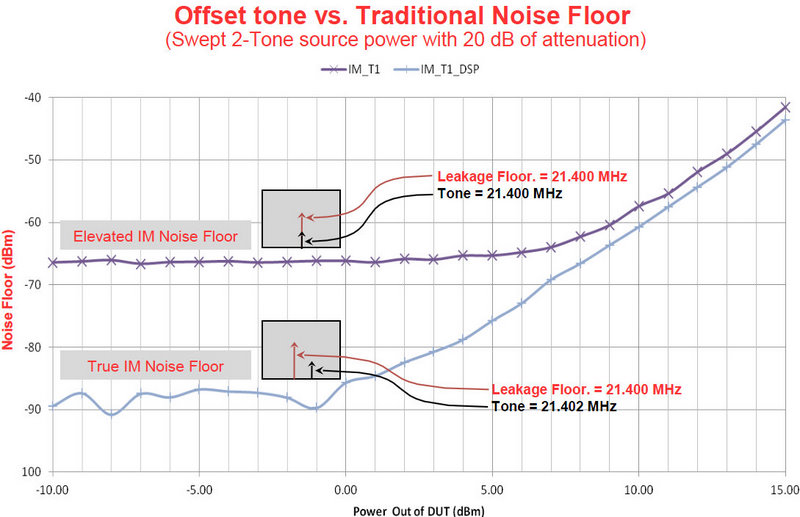When this same data is referenced to the main tone in the IM measurement, the actual SFDR of the measurement is the result. In this case the effective C/I range of the system went from less than 70 dB to more than 80.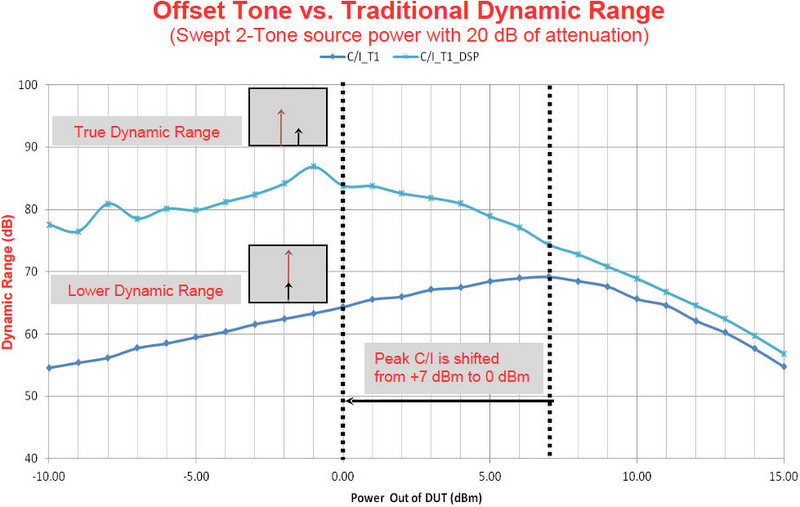https://roos.com/docs/MFEY-8PL2ZK
ROOS INSTRUMENTS CONFIDENTIAL AND PROPRIETARY